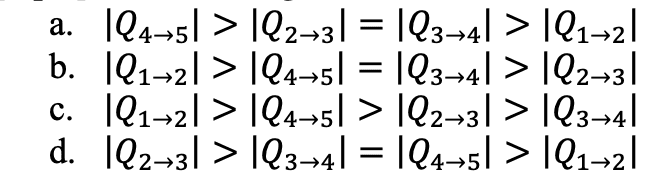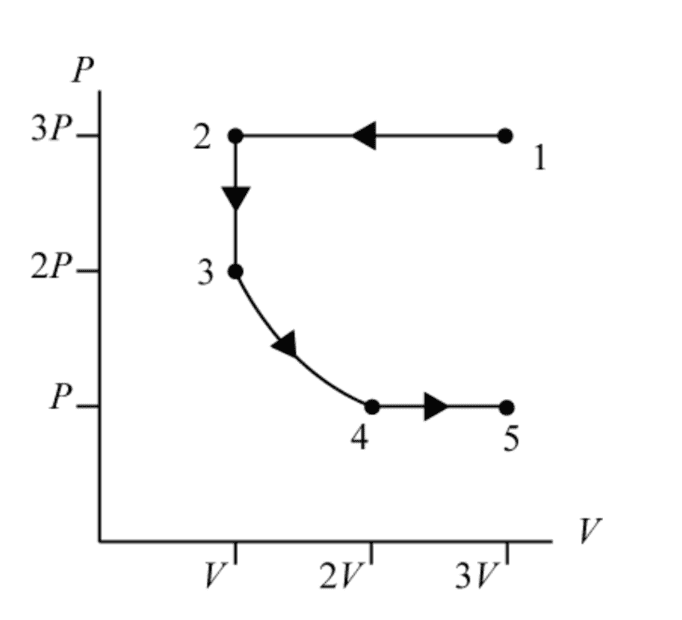# pV diagram, ranking heat transfer between 4 processes

HoboBones
Homework Statement:
Rank the magnitude of the heat transferred with the gas in each of the four processes.
Relevant Equations:
First law of thermodynamics
Thermal energy
Ideal gas law
Work done by gas
Work in isothermal

Last edited:

HoboBones
Problem and pV diagram

Problem: Rank the magnitude of the heat transferred with the gas in each of the four processes.Given pV diagramAttempt at solution with my questions in red

Equations used:

Thermal energy: Eth=3/2nRT
Ideal gas law: pV=nRT
Workby gas=area under curve
Work in isothermal: Wgas,isothermal=nRTln(Vf/Vi)
First law of thermodynamics applied to gases: ΔEth=Q-Wgas

Set up:

From ideal gas law, pV=nRT. Thus,

ΔEth=3/2nRT=3/2pV
Wgas, isothermal=nRTln(Vf/Vi)=pVln(Vf/Vi)
ΔEth=Q-Wgas --> Q=ΔEth+Wgas

Solving for ΔEth, Wgas, and |Q| for the processes: (I don't understand why we are solving for absolute value of Q)

I need some help solving for ΔE, Wgas, and |Q| for process 3->4

 Process​ ΔEth​ Wgas​ |Q|​ 1->2​ -9pV​ -6pV​ 15pV​ 2->3​ 3/2(-pV)​ 0​ 1.5pV​ 3->4​ 0pV​ ?​ ?​ 4->5​ 3/2(pV)​ pV​ 2.5pV​

Here is my attempt at process 3->4

ΔEth3->4 = 3/2(2pV-2pV) = 0 (My professor has the answer as "0pV" and not 0, not sure why?

Wgas,isothermal= area under the curve?

Area of triangle + Area under rectangle = 2pV? (correct answer is -pVln(Vf/Vi)

|Q| = -pVln(2V/V) = 1.4pV (I am not sure how this is the correct answer)

Any help would be much appreciated!

#### Attachments

Homework Helper
Gold Member
##dW=pdV.## Replace ##p## using the ideal gas law and integrate. Note that "isothermal" means "constant ##T##."

HoboBones
Update, I think I figured it out but not really understanding

For process 3->4,

Wgas,isothermal = area under curve = 1/2bh + Arectangle = 2pV

We can plug in our area into the work in isothermal equation, thus

Wgas,isothermal = -pVln(Vf/Vi) = -2pVln(2V/V)

|Q| = ΔEth(3->4) + Wgas,isothermal = 0pV -2pVln(2V/V) = |-1.386pV| = 1.4pV

HoboBones
##dW=pdV.## Replace ##p## using the ideal gas law and integrate. Note that "isothermal" means "constant ##T##."
She doesn't want us to use integrals unfortunately